SLVSAP2C December   2010  – February 2016

PRODUCTION DATA.

1. Features
2. Applications
3. Description
4. Revision History
5. Pin Configuration and Functions
6. Specifications
7. Detailed Description
8. Application and Implementation
1. 8.1 Application Information
2. 8.2 Typical Application
1. 8.2.1 Design Requirements
2. 8.2.2 Detailed Design Procedure
3. 8.2.3 Application Curves
9. Power Supply Recommendations
10. 10Layout
11. 11Device and Documentation Support
1. 11.1 Device Support
2. 11.2 Documentation Support
3. 11.3 Community Resource
4. 11.4 Trademarks
5. 11.5 Electrostatic Discharge Caution
6. 11.6 Glossary
12. 12Mechanical, Packaging, and Orderable Information

• DGQ|10
• DRC|10
• DGQ|10
• DRC|10

## 7 Detailed Description

### 7.1 Overview

The TPS57060-Q1 device is a 60-V, 0.5-A, step-down (buck) regulator with an integrated high side n-channel MOSFET. To improve performance during line and load transients the device implements a constant frequency, current mode control which reduces output capacitance and simplifies external frequency compensation design. The wide switching frequency of 100 kHz to 2500 kHz allows for efficiency and size optimization when selecting the output filter components. A resistor to ground on the RT/CLK pin sets the switching frequency. The device has an internal phase lock loop (PLL) on the RT/CLK pin that is used to synchronize the power switch turn on to a falling edge of an external system clock.

The TPS57060-Q1 device has a default start up voltage of approximately 2.5 V. The EN pin has an internal pullup current source that can be used to adjust the input voltage under voltage lockout (UVLO) threshold with two external resistors. In addition, the pullup current provides a default condition. When the EN pin is floating the device will operate. The operating current is 116 μA when not switching and under no load. When the device is disabled, the supply current is 1.3 μA.

The integrated 200-mΩ high-side MOSFET allows for high efficiency power supply designs capable of delivering 0.5 amperes of continuous current to a load. The TPS57060-Q1 device reduces the external component count by integrating the boot recharge diode. A capacitor between the BOOT and PH pins supplies the bias voltage for the integrated high-side MOSFET. The boot capacitor voltage is monitored by an UVLO circuit and will turn the high-side MOSFET off when the boot voltage falls below a preset threshold. The TPS57060-Q1 device can operate at high duty cycles because of the boot UVLO. The output voltage can be stepped down to as low as the 0.8-V reference.

The TPS57060-Q1 device has a power good comparator (PWRGD) which asserts when the regulated output voltage is less than 92% or greater than 109% of the nominal output voltage. The PWRGD pin is an open drain output which deasserts when the VSENSE pin voltage is between 94% and 107% of the nominal output voltage allowing the pin to transition high when a pullup resistor is used.

The TPS57060-Q1 device minimizes excessive output overvoltage (OV) transients by taking advantage of the OV power good comparator. When the OV comparator is activated, the high-side MOSFET is turned off and masked from turning on until the output voltage is lower than 107%.

The SS/TR (slow start/tracking) pin minimizes inrush currents and provides power-supply sequencing during power up. A small value capacitor should be coupled to the pin to adjust the slow start time. A resistor divider can be coupled to the pin for critical power supply sequencing requirements. The SS/TR pin is discharged before the output powers up. This discharging ensures a repeatable restart after an over-temperature fault, UVLO fault or a disabled condition.

The TPS57060-Q1 device also discharges the slow start capacitor during overload conditions with an overload recovery circuit. The overload recovery circuit will slow start the output from the fault voltage to the nominal regulation voltage when a fault condition is removed. A frequency foldback circuit reduces the switching frequency during startup and overcurrent fault conditions to help control the inductor current.

### 7.2 Functional Block Diagram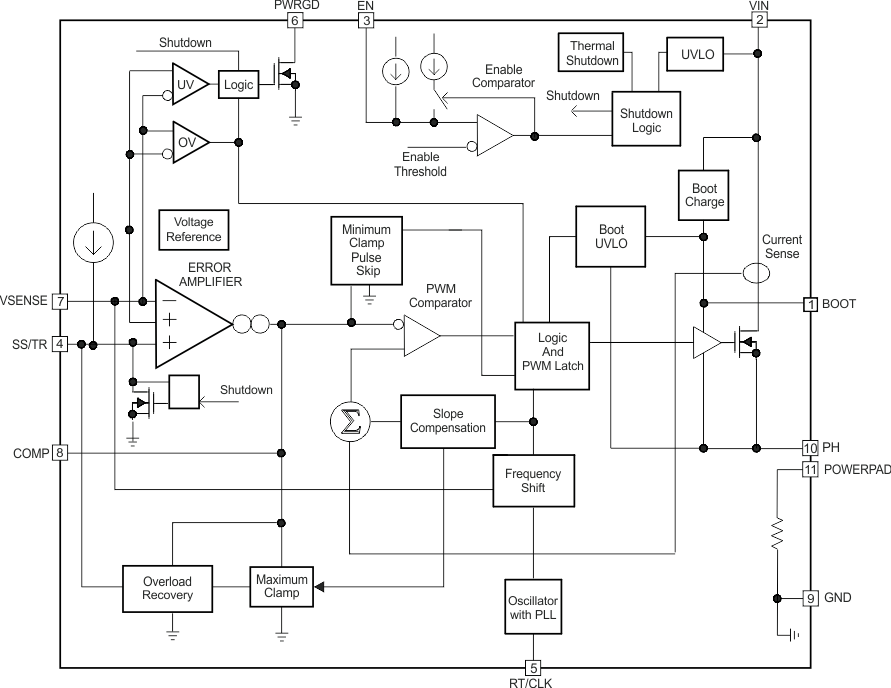### 7.3 Feature Description

#### 7.3.1 Fixed Frequency PWM Control

The TPS57060-Q1 device uses an adjustable fixed frequency, peak current mode control. The output voltage is scaled downed to the internal voltage reference level using the external voltage divider resistors on the VSENSE pin and this voltage is compared to an internal voltage reference by an error amplifier which drives the COMP pin. An internal oscillator initiates the turn on of the high-side power switch. The error amplifier output is compared to the high-side power-switch current. When the power switch current reaches the level set by the COMP voltage, the power switch is turned off. The COMP pin voltage will increase and decrease as the output current increases and decreases. The device implements a current limit by clamping the COMP pin voltage to a maximum level. The Eco-Mode™ is implemented with a minimum clamp on the COMP pin.

#### 7.3.2 Slope Compensation Output Current

The TPS57060-Q1 device adds a compensating ramp to the switch current signal. This slope compensation prevents sub-harmonic oscillations. The available peak inductor current remains constant over the full duty-cycle range.

#### 7.3.3 Low Dropout Operation and Bootstrap Voltage (BOOT)

The TPS57060-Q1 device has an integrated boot regulator, and requires a small ceramic capacitor between the BOOT and PH pins to provide the gate drive voltage for the high-side MOSFET. The BOOT capacitor is refreshed when the high-side MOSFET is off and the low-side diode conducts. The value of this ceramic capacitor should be 0.1 μF. A ceramic capacitor with an X7R or X5R grade dielectric with a voltage rating of 10 V or higher is recommended because of the stable characteristics overtemperature and voltage.

To improve drop out, the TPS57060-Q1 device is designed to operate at 100% duty cycle as long as the BOOT to PH pin voltage is greater than 2.1 V. When the voltage from BOOT to PH drops below 2.1 V, the high-side MOSFET is turned off using an UVLO circuit which allows the low-side diode to conduct and refresh the charge on the BOOT capacitor. Because the supply current sourced from the BOOT capacitor is low, the high-side MOSFET can remain on for more switching cycles than are required to refresh the capacitor, thus the effective duty cycle of the switching regulator is high.

The effective duty cycle during dropout of the regulator is mainly influenced by the voltage drops across the power MOSFET, inductor resistance, low-side diode and printed circuit board resistance. During operating conditions in which the input voltage drops and the regulator is operating in continuous conduction mode, the high-side MOSFET can remain on for 100% of the duty cycle to maintain output regulation, until the BOOT to PH voltage falls below 2.1 V.

Attention must be taken in maximum duty cycle applications which experience extended time periods with light loads or no load. When the voltage across the BOOT capacitor falls below the 2.1-V UVLO threshold, the high-side MOSFET is turned off, but there may not be enough inductor current to pull the PH pin down to recharge the BOOT capacitor. The high-side MOSFET of the regulator stops switching because the voltage across the BOOT capacitor is less than 2.1 V. The output capacitor then decays until the difference in the input voltage and output voltage is greater than 2.1 V, at which point the BOOT UVLO threshold is exceeded, and the device starts switching again until the desired output voltage is reached. This operating condition persists until the input voltage, the load current increases, or both. TI recommends to adjust the VIN stop voltage greater than the BOOT UVLO trigger condition at the minimum load of the application using the adjustable VIN UVLO feature with resistors on the EN pin.

The start and stop voltages for typical 3.3-V and 5-V output applications are shown in Figure 25 and Figure 26. The voltages are plotted versus load current. The start voltage is defined as the input voltage needed to regulate the output within 1%. The stop voltage is defined as the input voltage at which the output drops by 5% or stops switching.

During high duty cycle conditions, the inductor current ripple increases while the BOOT capacitor is being recharged resulting in an increase in ripple voltage on the output. This is due to the recharge time of the boot capacitor being longer than the typical high side off time when switching occurs every cycle.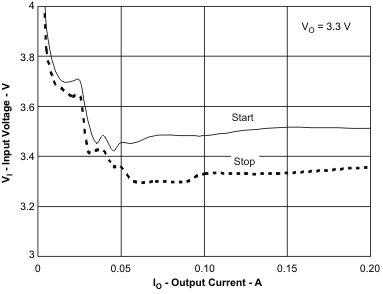Figure 25. 3.3-V Start and Stop Voltage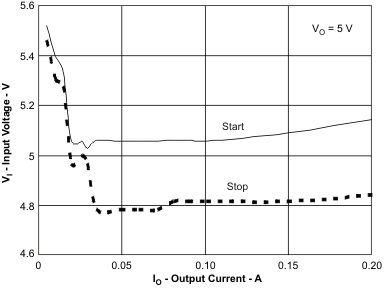Figure 26. 5-V Start and Stop Voltage

#### 7.3.4 Error Amplifier

The TPS57060-Q1 device has a transconductance amplifier for the error amplifier. The error amplifier compares the VSENSE voltage to the lower of the SS/TR pin voltage or the internal 0.8-V voltage reference. The transconductance (gm) of the error amplifier is 97 μA/V during normal operation. During the slow start operation, the transconductance is a fraction of the normal operating gm. When the voltage of the VSENSE pin is below 0.8 V and the device is regulating using the SS/TR voltage, the gm is 25 μA/V.

The frequency compensation components (capacitor, series resistor and capacitor) are added to the COMP pin to ground.

#### 7.3.5 Voltage Reference

The voltage reference system produces a precise ±2% voltage reference over temperature by scaling the output of a temperature stable bandgap circuit.

#### 7.3.6 Adjusting the Output Voltage

The output voltage is set with a resistor divider from the output node to the VSENSE pin. TI recommends to use 1% tolerance or better divider resistors. Refer to the schematic in Figure 40, start with 10 kΩ for the R2 resistor and use Equation 1 to calculate R1. To improve efficiency at light loads consider using larger value resistors. If the values are too high the regulator will be more susceptible to noise and voltage errors from the VSENSE input current will be noticeable

Equation 1.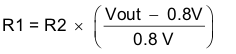#### 7.3.7 Enable and Adjusting Undervoltage Lockout (UVLO)

The TPS57060-Q1 device is disabled when the VIN pin voltage falls below 2.5 V. If an application requires a higher undervoltage lockout (UVLO), use the EN pin as shown in Figure 27 to adjust the input voltage UVLO by using the two external resistors. Although using the UVLO adjust registers is not is not required, consistent power-up behavior is highly recommended for operation. The EN pin has an internal pullup current source, I1, of 0.9 μA that provides the default condition of the TPS57060-Q1 device operating when the EN pin floats. When the EN pin voltage exceeds 1.25 V, an additional 2.9 μA of hysteresis, Ihys, is added. This additional current facilitates input voltage hysteresis. Use Equation 2 to set the external hysteresis for the input voltage. Use Equation 3 to set the input start voltage.Figure 27. Adjustable Undervoltage Lockout (UVLO)
Equation 2.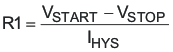Equation 3.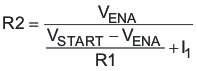Another technique to add input voltage hysteresis is shown in Figure 28. This method may be used, if the resistance values are high from the previous method and a wider voltage hysteresis is needed. The resistor R3 sources additional hysteresis current into the EN pin.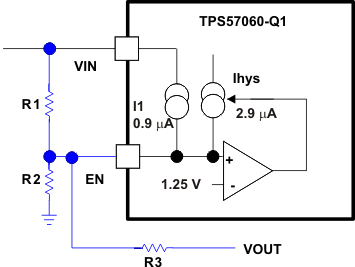Figure 28. Adding Additional Hysteresis
Equation 4.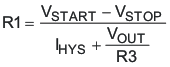Equation 5.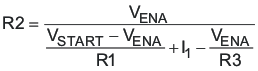Do not place a low-impedance voltage source with greater than 5 V directly on the EN pin. Do not place a capacitor directly on the EN pin if VEN > 5 V when using a voltage divider to adjust the start and stop voltage. The node voltage, (see Figure 29) must remain equal to or less than 5.8 V. The zener diode can sink up to 100 μA. The EN pin voltage can be greater than 5 V if the VIN voltage source has a high impedance and does not source more than 100 μA into the EN pin.Figure 29. Node Voltage

#### 7.3.8 Slow Start and Tracking Pin (SS/TR)

The TPS57060-Q1 device effectively uses the lower voltage of the internal voltage reference or the SS/TR pin voltage as the reference voltage of the power supply and regulates the output accordingly. A capacitor on the SS/TR pin to ground implements a slow start time. The TPS57060-Q1 device has an internal pullup current source of 2 μA that charges the external slow-start capacitor. The calculations for the slow start time (10% to 90%) are shown in Equation 6. The voltage reference (VREF) is 0.8 V and the slow start current (ISS) is 2μA. The slow start capacitor should remain lower than 0.47 μF and greater than 0.47 nF.

Equation 6.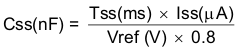At power up, the TPS57060-Q1 device does not start switching until the slow start pin is discharged to less than 40 mV to ensure a proper power up, see Figure 30.

Also, during normal operation, the TPS57060-Q1 device stops switching and the SS/TR must be discharged to 40 mV, when the VIN UVLO is exceeded, EN pin pulled below 1.25 V, or a thermal shutdown event occurs.

The VSENSE voltage will follow the SS/TR pin voltage with a 45-mV offset up to 85% of the internal voltage reference. When the SS/TR voltage is greater than 85% on the internal reference voltage the offset increases as the effective system reference transitions from the SS/TR voltage to the internal voltage reference (see Figure 23). The SS/TR voltage will ramp linearly until clamped at 1.7 V.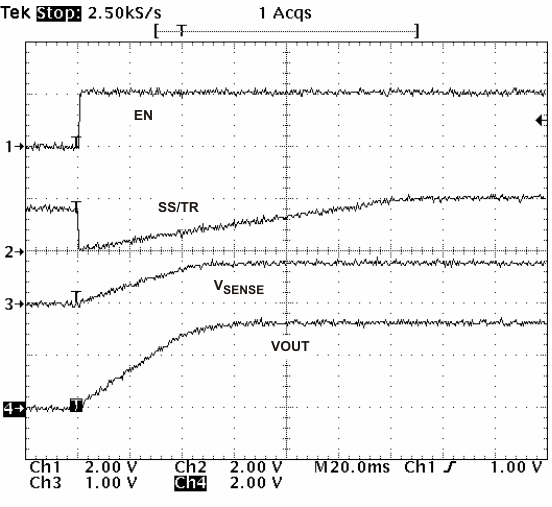Figure 30. Operation of SS/TR Pin When Starting

#### 7.3.9 Overload Recovery Circuit

The TPS57060-Q1 has an overload recovery (OLR) circuit. The OLR circuit will slow start the output from the overload voltage to the nominal regulation voltage when the fault condition is removed. The OLR circuit will discharge the SS/TR pin to a voltage slightly greater than the VSENSE pin voltage using an internal pulldown of 100μA when the error amplifier is changed to a high voltage from a fault condition. When the fault condition is removed, the output will slow start from the fault voltage to nominal output voltage.

#### 7.3.10 Constant Switching Frequency and Timing Resistor (RT/CLK Pin)

The switching frequency of the TPS57060-Q1 device is adjustable over a wide range from approximately 100 kHz to 2500 kHz by placing a resistor on the RT/CLK pin. The RT/CLK pin voltage is typically 0.5 V and must have a resistor to ground to set the switching frequency. To determine the timing resistance for a given switching frequency, use Equation 7 or the curves in Figure 31 or Figure 32. To reduce the solution size, set the switching frequency as high as possible, but tradeoffs of the supply efficiency, maximum input voltage and minimum controllable on time should be considered.

The minimum controllable on time is typically 130 ns and limits the maximum operating input voltage.

The maximum switching frequency is also limited by the frequency shift circuit. Use Equation 7 or the curves in Figure 31 or Figure 32 to calculate the timing resistor values for the required switching frequency.

Equation 7.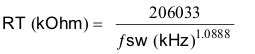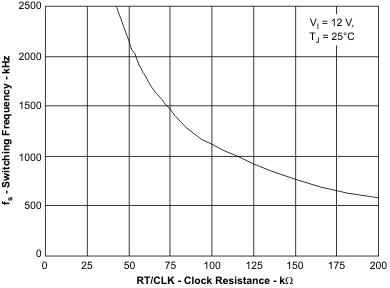Figure 31. High Range RT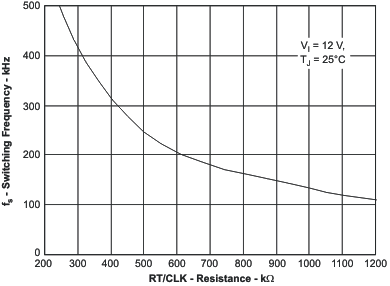Figure 32. Low Range RT

#### 7.3.11 Overcurrent Protection and Frequency Shift

The TPS57060-Q1 device implements current mode control which uses the COMP pin voltage to turn off the high-side MOSFET on a cycle-by-cycle basis. Each cycle the switch current and COMP pin voltage are compared, when the peak switch current intersects the COMP voltage, the high side switch is turned off. During overcurrent conditions that pull the output voltage low, the error amplifier responds by driving the COMP pin high, increasing the switch current. The error amplifier output is clamped internally, which functions as a switch current limit.

To increase the maximum operating switching frequency at high input voltages the TPS57060-Q1 device implements a frequency shift. The switching frequency is divided by 8, 4, 2, and 1 as the voltage ramps from 0 to 0.8 vV on VSENSE pin.

The device implements a digital frequency shift to enable synchronizing to an external clock during normal startup and fault conditions. Because the device can only divide the switching frequency by 8, the device operates within a maximum input voltage limit that still allows for frequency shift protection.

During short-circuit events (particularly with high input voltage applications), the control loop has a finite minimum controllable on time and the output has a low voltage. During the switch on time, the inductor current ramps to the peak current limit because of the high input voltage and minimum on time. During the switch off time, the inductor would normally not have enough off time and output voltage for the inductor to ramp down by the ramp up amount. The frequency shift effectively increases the off time allowing the current to ramp down.

#### 7.3.12 Selecting the Switching Frequency

The switching frequency that is selected should be the lower value of the two equations, Equation 8 and Equation 9. Equation 8 is the maximum switching frequency limitation set by the minimum controllable on time. Setting the switching frequency above this value will cause the regulator to skip switching pulses.

Equation 9 is the maximum switching frequency limit set by the frequency shift protection. To have adequate output short circuit protection at high input voltages, the switching frequency should be set to be less than the fsw(maxshift) frequency. In Equation 9, to calculate the maximum switching frequency one must take into account that the output voltage decreases from the nominal voltage to 0 V, the fdiv integer increases from 1 to 8 corresponding to the frequency shift.

In Figure 33, the solid line illustrates a typical safe operating area regarding frequency shift and assumes the output voltage is zero volts, and the resistance of the inductor is 0.130 Ω, FET on resistance of 0.2 Ω and the diode voltage drop is 0.5 V. The dashed line is the maximum switching frequency to avoid pulse skipping. Enter these equations in a spreadsheet or other software or use the SwitcherPro design software to determine the switching frequency.

Equation 8.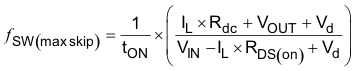Equation 9.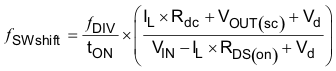where

• IL = Inductor current
• Rdc = Inductor resistance
• VIN = Maximum input voltage
• VOUT = Output voltage
• VOUTSC = Output voltage during short
• Vd = Diode voltage drop
• rDS(on) = Switch on-resistance
• tON = Controllable on-time
• ƒDIV = Frequency divide (equals 1, 2, 4, or 8)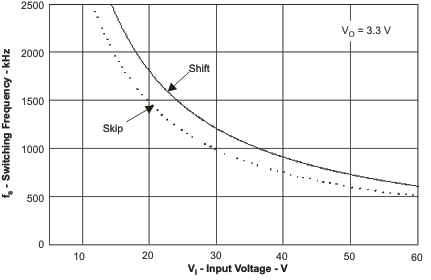Figure 33. Maximum Switching Frequency vs. Input Voltage

#### 7.3.13 How to Interface to RT/CLK Pin

The RT/CLK pin can be used to synchronize the regulator to an external system clock. To implement the synchronization feature connect a square wave to the RT/CLK pin through the circuit network shown in Figure 34. The square wave amplitude must transition lower than 0.5 V and higher than 2.2 V on the RT/CLK pin and have an on time greater than 40 ns and an off time greater than 40 ns. The synchronization frequency range is 300 kHz to 2200 kHz. The rising edge of the PH will be synchronized to the falling edge of RT/CLK pin signal. The external synchronization circuit should be designed in such a way that the device will have the default frequency set resistor connected from the RT/CLK pin to ground should the synchronization signal turn off. TI recommends to use a frequency set resistor connected as shown in Figure 34 through a 50-Ω resistor to ground. The resistor should set the switching frequency close to the external CLK frequency. TI recommends to AC couple the synchronization signal through a 10-pF ceramic capacitor to RT/CLK pin and a 4-kΩ series resistor. The series resistor reduces PH jitter in heavy load applications when synchronizing to an external clock and in applications which transition from synchronizing to RT mode. The first time the CLK is pulled above the CLK threshold the device switches from the RT resistor frequency to PLL mode. The internal 0.5-V voltage source is removed and the CLK pin becomes high impedance as the PLL starts to lock onto the external signal. Because there is a PLL on the regulator the switching frequency can be higher or lower than the frequency set with the external resistor. The device transitions from the resistor mode to the PLL mode and then will increase or decrease the switching frequency until the PLL locks onto the CLK frequency within 100 ms.

When the device transitions from the PLL to resistor mode the switching frequency will slow down from the CLK frequency to 150 kHz, then reapply the 0.5-V voltage and the resistor then sets the switching frequency. The switching frequency is divided by 8, 4, 2, and 1 as the voltage ramps from 0 to 0.8 V on VSENSE pin. The device implements a digital frequency shift to enable synchronizing to an external clock during normal startup and fault conditions. Figure 35, Figure 36 and Figure 37 show the device synchronized to an external system clock in continuous conduction mode (CCM) discontinuous conduction (DCM) and pulse skip mode (PSM).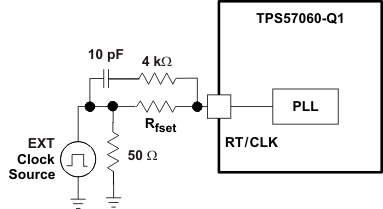Figure 34. Synchronizing to a System Clock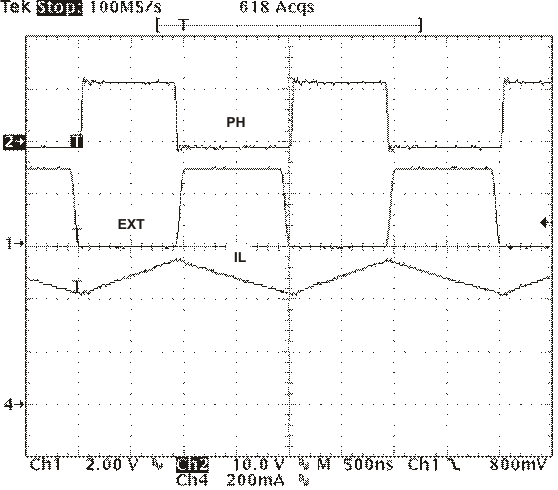Figure 35. Plot of Synchronizing in CCM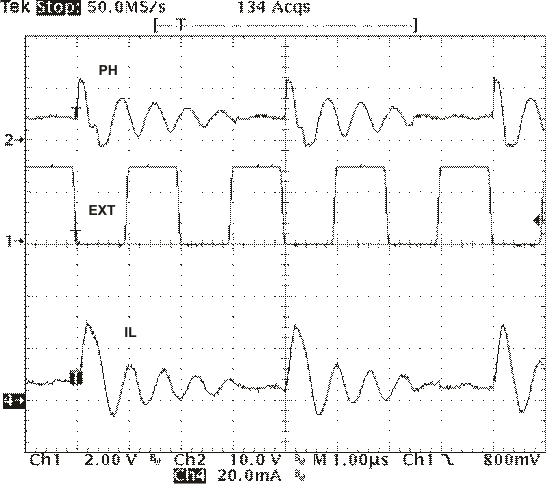Figure 37. Plot of Synchronizing in PSM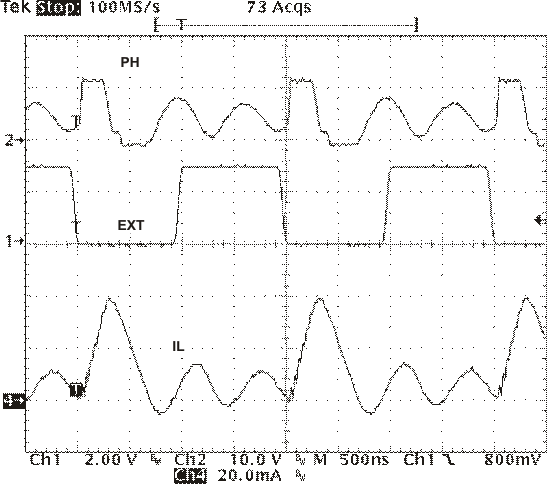Figure 36. Plot of Synchronizing in DCM

#### 7.3.14 Power Good (PWRGD Pin)

The PWRGD pin is an open drain output. When the VSENSE pin is between 94% and 107% of the internal voltage reference the PWRGD pin is de-asserted and the pin floats. TI recommends to use a pullup resistor between the values of 1 kΩ and 100 kΩ to a voltage source that is 5.5 V or less. The PWRGD is in a defined state when the VIN input voltage is greater than 1.5 V but with reduced current sinking capability. The PWRGD achieves full current sinking capability as VIN input voltage approaches 3 V.

The PWRGD pin is pulled low when the VSENSE is lower than 92% or greater than 109% of the nominal internal reference voltage. Also, the PWRGD is pulled low, if the UVLO or thermal shutdown are asserted or the EN pin pulled low.

#### 7.3.15 Overvoltage Transient Protection

The TPS57060-Q1 device incorporates an overvoltage transient protection (OVTP) circuit to minimize voltage overshoot when recovering from output fault conditions or strong unload transients on power supply designs with low value output capacitance. For example, when the power supply output is overloaded the error amplifier compares the actual output voltage to the internal reference voltage. If the VSENSE pin voltage is lower than the internal reference voltage for a considerable time, the output of the error amplifier will respond by clamping the error amplifier output to a high voltage. Thus, requesting the maximum output current. When the condition is removed, the regulator output rises and the error amplifier output transitions to the steady state duty cycle. In some applications, the power supply output voltage can respond faster than the error amplifier output can respond, this actuality leads to the possibility of an output overshoot. The OVTP feature minimizes the output overshoot, when using a low value output capacitor, by implementing a circuit to compare the VSENSE pin voltage to OVTP threshold which is 109% of the internal voltage reference. If the VSENSE pin voltage is greater than the OVTP threshold, the high-side MOSFET is disabled preventing current from flowing to the output and minimizing output overshoot. When the VSENSE voltage drops lower than the OVTP threshold, the high-side MOSFET is allowed to turn on at the next clock cycle.

#### 7.3.16 Thermal Shutdown

The device implements an internal thermal shutdown to protect itself if the junction temperature exceeds 182°C. The thermal shutdown forces the device to stop switching when the junction temperature exceeds the thermal trip threshold. When the die temperature decreases below 182°C, the device reinitiates the power up sequence by discharging the SS/TR pin.

#### 7.3.17 Small Signal Model for Loop Response

Figure 38 shows an equivalent model for the TPS57060-Q1 control loop which can be modeled in a circuit simulation program to check frequency response and dynamic load response. The error amplifier is a transconductance amplifier with a gmEA of 97 μA/V. The error amplifier can be modeled using an ideal voltage controlled current source. The resistor Ro and capacitor Co model the open loop gain and frequency response of the amplifier. The 1-mV AC voltage source between the nodes a and b effectively breaks the control loop for the frequency response measurements. Plotting c/a shows the small signal response of the frequency compensation. Plotting a/b shows the small signal response of the overall loop. The dynamic loop response can be checked by replacing RL with a current source with the appropriate load step amplitude and step rate in a time domain analysis. This equivalent model is only valid for continuous conduction mode designs.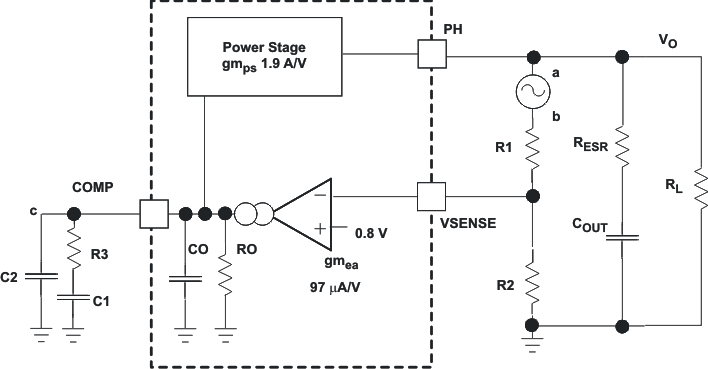Figure 38. Small Signal Model for Loop Response

#### 7.3.18 Simple Small-Signal Model for Peak Current-Mode Control

Figure 39 shows a simple small signal model that can be used to understand how to design the frequency compensation. The TPS57060-Q1 power stage can be approximated to a voltage-controlled current source (duty cycle modulator) supplying current to the output capacitor and load resistor. The control to output transfer function is shown in Equation 10 and consists of a DC gain, one dominant pole, and one ESR zero. The quotient of the change in switch current and the change in COMP pin voltage (node c in Figure 38) is the power stage transconductance. The gmPS for the TPS57060-Q1 device is 1.9 A/V. The low-frequency gain of the power stage frequency response is the product of the transconductance and the load resistance as shown in Equation 11.

As the load current increases and decreases, the low-frequency gain decreases and increases, respectively. This variation with the load may seem problematic at first glance, but fortunately the dominant pole moves with the load current (see Equation 12). The combined effect is highlighted by the dashed line in the right half of Figure 39. As the load current decreases, the gain increases and the pole frequency lowers, keeping the 0-dB crossover frequency the same for the varying load conditions which makes it easier to design the frequency compensation. The type of output capacitor chosen determines whether the ESR zero has a profound effect on the frequency compensation design. Using high ESR aluminum electrolytic capacitors may reduce the number frequency compensation components needed to stabilize the overall loop because the phase margin increases from the ESR zero at the lower frequencies (see Table 1).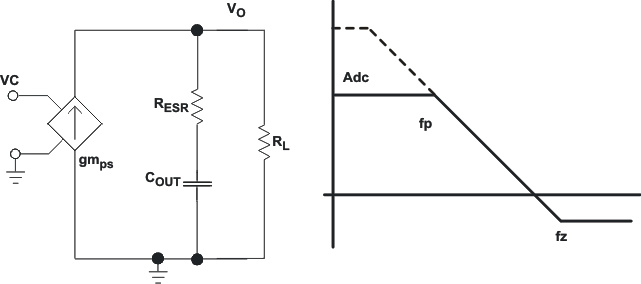Figure 39. Simple Small-Signal Model and Frequency Response for Peak Current-Mode Control
Equation 10.Equation 11.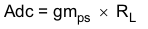Equation 12.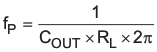Equation 13.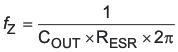#### 7.3.19 Small Signal Model for Frequency Compensation

The TPS57060-Q1 device uses a transconductance amplifier for the error amplifier and readily supports three of the commonly-used frequency compensation circuits. Compensation circuits Type 2A, Type 2B, and Type 1 are shown in Figure 40. Type 2 circuits most likely implemented in high bandwidth power-supply designs using low ESR output capacitors. The Type 1 circuit is used with power-supply designs with high-ESR aluminum electrolytic or tantalum capacitors.. Equation 14 and Equation 15 show how to relate the frequency response of the amplifier to the small signal model in Figure 40. The open-loop gain and bandwidth are modeled using the RO and CO shown in Figure 40. See the application section for a design example using a Type 2A network with a low ESR output capacitor.

Equation 14 through Equation 23 are provided as a reference for those who prefer to compensate using the preferred methods. Those who prefer to use prescribed method use the method outlined in the application section or use switched information.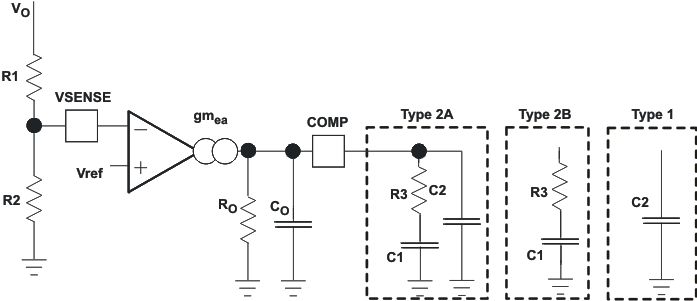Figure 40. Types of Frequency Compensation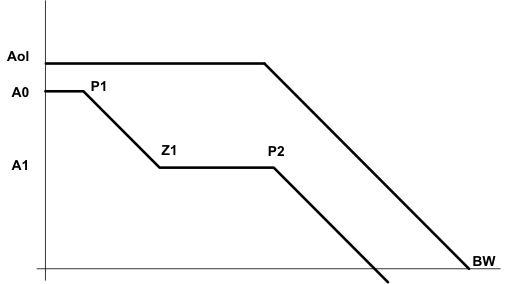Figure 41. Frequency Response of the Type 2A and Type 2B Frequency Compensation
Equation 14.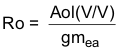Equation 15.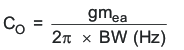Equation 16.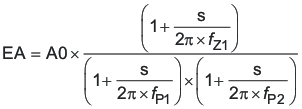Equation 17.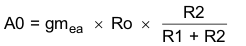Equation 18.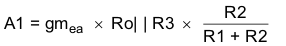Equation 19.Equation 20.Equation 21.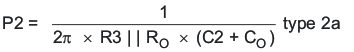Equation 22.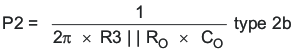Equation 23.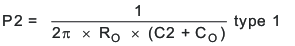### 7.4 Device Functional Modes

#### 7.4.1 Sequencing

Many of the common power supply sequencing methods can be implemented using the SS/TR, EN and PWRGD pins. The sequential method can be implemented using an open drain output of a power on reset pin of another device. The sequential method is illustrated in Figure 42 using two TPS57060-Q1 devices. The power good is coupled to the EN pin on the TPS57060-Q1 device which enables the second power supply when the primary supply reaches regulation. If needed, a 1nF ceramic capacitor on the EN pin of the second power supply provides a 1-ms start-up delay. Figure 43 shows the results of Figure 42.

Figure 44 shows a method for ratiometric start-up sequence by connecting the SS/TR pins together. The regulator outputs will ramp up and reach regulation at the same time. When calculating the slow start time the pullup current source must be doubled in Equation 6. Figure 45 shows the results of Figure 44.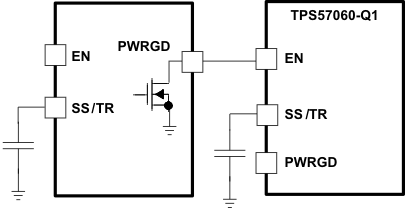Figure 42. Schematic for Sequential Start-Up Sequence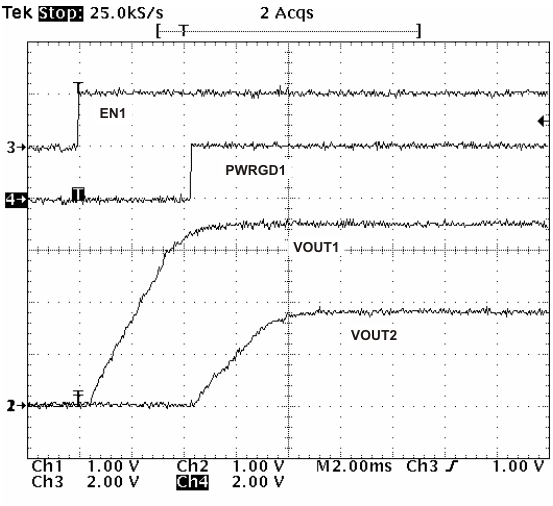Figure 43. Sequential Startup Using EN and PWRGD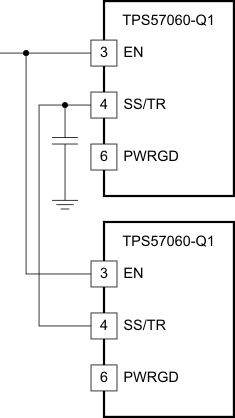Figure 44. Schematic for Ratiometric Start-Up Using Coupled SS/TR Pins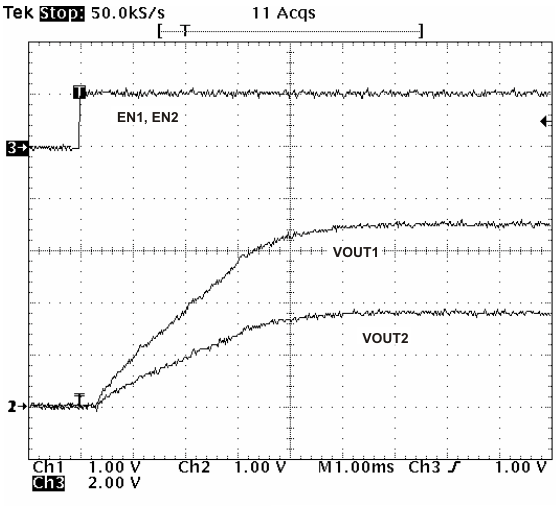Figure 45. Ratiometric Startup Using Coupled SS/TR pins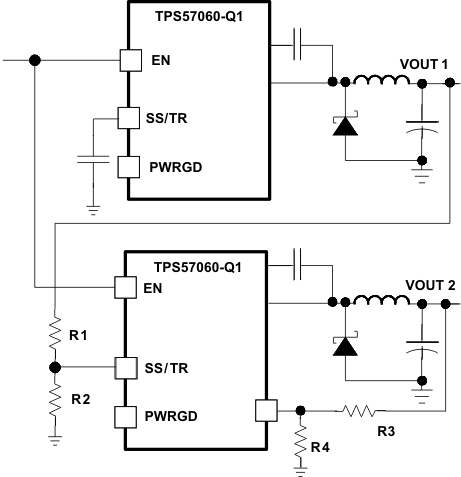Figure 46. Schematic for Ratiometric and Simultaneous Start-Up Sequence

Ratiometric and simultaneous power supply sequencing can be implemented by connecting the resistor network of R1 and R2 shown in Figure 46 to the output of the power supply that needs to be tracked or another voltage reference source. Using Equation 24 and Equation 25, the tracking resistors can be calculated to initiate the Vout2 slightly before, after or at the same time as Vout1. Equation 26 is the voltage difference between Vout1 and Vout2 at the 95% of nominal output regulation.

The deltaV variable is 0 V for simultaneous sequencing. To minimize the effect of the inherent SS/TR to VSENSE offset (Vssoffset) in the slow start circuit and the offset created by the pullup current source (Iss) and tracking resistors, the Vssoffset and Iss are included as variables in the equations.

To design a ratiometric start up in which the Vout2 voltage is slightly greater than the Vout1 voltage when Vout2 reaches regulation, use a negative number in Equation 24 through Equation 26 for deltaV. Equation 26 results in a positive number for applications which the Vout2 is slightly lower than Vout1 when Vout2 regulation is achieved.

Because the SS/TR pin must be pulled below 40 mV before starting after an EN, UVLO or thermal shutdown fault, careful selection of the tracking resistors is needed to ensure the device will restart after a fault. Make sure the calculated R1 value from Equation 24 is greater than the value calculated in Equation 27 to ensure the device can recover from a fault.

As the SS/TR voltage becomes more than 85% of the nominal reference voltage the Vssoffset becomes larger as the slow start circuits gradually handoff the regulation reference to the internal voltage reference. The SS/TR pin voltage needs to be greater than 1.3 V for a complete handoff to the internal voltage reference as shown in Figure 23.

Equation 24.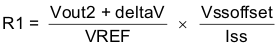Equation 25.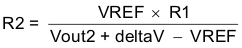Equation 26.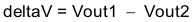Equation 27.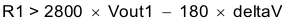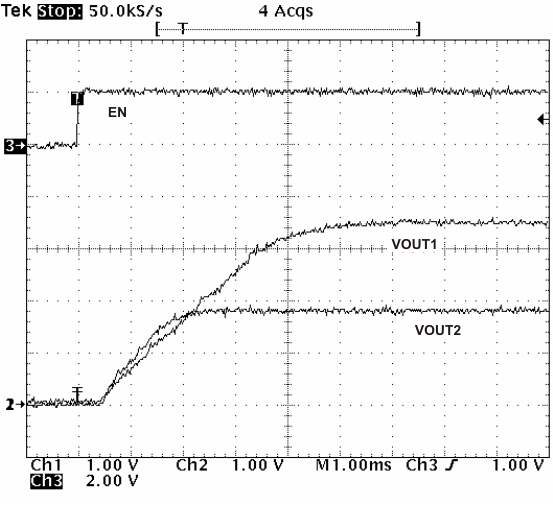Figure 47. Ratiometric Startup With VOUT2 Leading VOUT1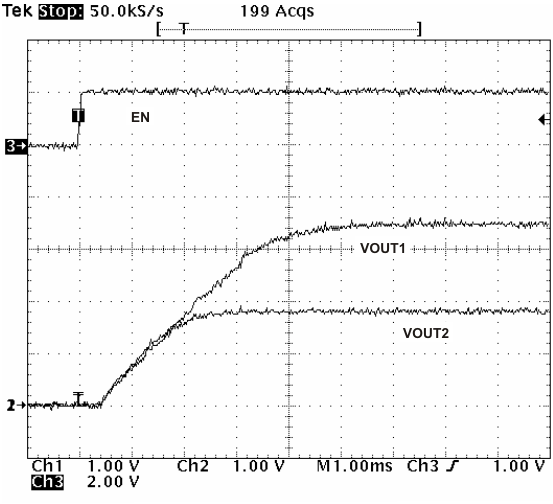Figure 49. Simultaneous Startup With Tracking Resistor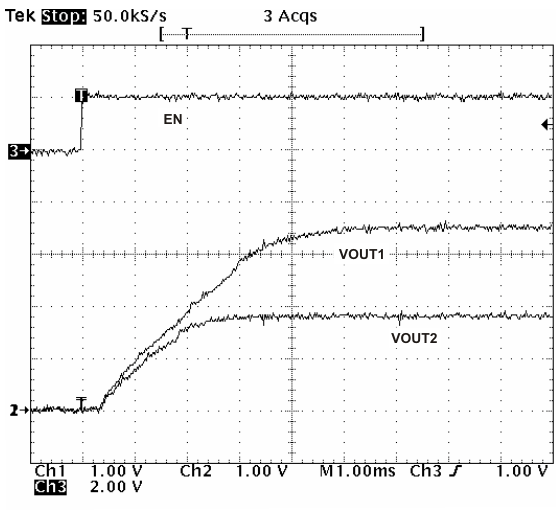Figure 48. Ratiometric Startup With VOUT1 Leading VOUT2

#### 7.4.2 Pulse Skip Eco-Mode

The TPS57060-Q1 device operates in a pulse-skip Eco mode at light load currents to improve efficiency by reducing switching and gate drive losses. The TPS57060-Q1 device is designed so that if the output voltage is within regulation and the peak switch current at the end of any switching cycle is below the pulse skipping current threshold, the device enters Eco mode. This current threshold is the current level corresponding to a nominal COMP voltage of 500 mV.

When in Eco-mode, the COMP pin voltage is clamped at 500 mV and the high-side MOSFET is inhibited. Further decreases in load current or in output voltage cannot drive the COMP pin below this clamp voltage level.

Because the device is not switching, the output voltage begins to decay. As the voltage control loop compensates for the falling output voltage, the COMP pin voltage begins to rise. At this time, the high-side MOSFET is enabled and a switching pulse initiates on the next switching cycle. The peak current is set by the COMP pin voltage. The output voltage re-charges the regulated value (see Figure 50), then the peak switch current starts to decrease, and eventually falls below the Eco mode threshold at which time the device again enters Eco mode.

For Eco mode operation, the TPS57060-Q1 device senses peak current, not average or load current, so the load current where the device enters Eco mode is dependent on the minimum on-time, input voltage, output voltage, and output inductance value. For example, the circuit in Figure 51 enters Eco mode at about 20 mA of output current. When the load current is low and the output voltage is within regulation, the device enters a sleep mode and draws only 116-μA input quiescent current. The internal PLL remains operating when in sleep mode. When operating at light load currents in the pulse skip mode, the switching transitions occur synchronously with the external clock signal.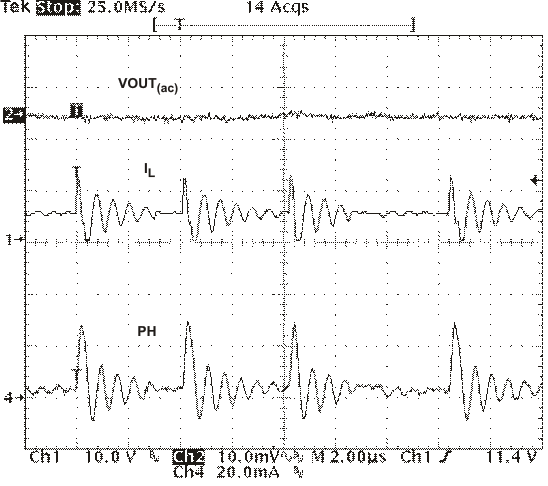Figure 50. Pulse-Skip Mode Operation